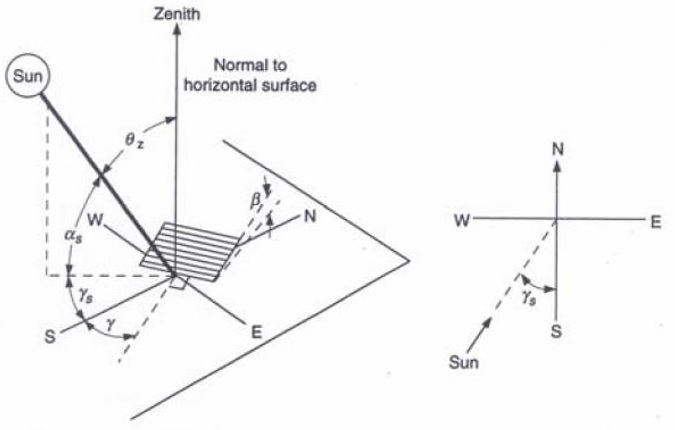# What is the optimal angle for maximizing solar gain?

Solar Energy is most effective and most concentrated when the position of the receiver is  normal to the incident rays. As the position of the sun in the sky keeps changing, the angle of the receiver and its orientation must also change for receiving maximum amount of radiation. Therefore a tracking system is needed that can change inclination as the sun climbs up/down the sky. An efficient tracking system must also have the ability to change the  azimuth of the receiver as the sun moves from east to west. Thus one, two and even three axis tracking systems are used on many commercial/ utility scale solar projects.

When Solar PV or water heating systems are installed for domestic applications, tracking systems are not used as they are expensive and at times impractical.  Solar installations on homes , barns and farms are mostly mounted at a fixed angle.  As they sit flush against the roof, their angle is the same as the roof pitch angle. The question is therefore, is the roof inclination the best angle for maximizing solar gain?

Roof pitches vary from country to country location to location. On the other hand, Solar insolation (incident solar radiation) at a particular location strongly varies with the latitude of that location.   It should be noted that both PV and Solar water heaters can last decades. Therefore the solar gain over life-time can be significantly reduced if the angle of installation is away from the optimal angle.

## Angle for Maximizing Solar Gain

There is a  thumb rule that is used  very commonly in the solar industry. An angle that equals 0.9 times the latitude (Φ) of the location (where PV or water heater needs to be installed) normally provides a value that is close to the optimal angle. To work out the optimal angle accurately, complex calculation is required that involves the use of hourly data measured for the site for a duration of an year.Left: Azimuth angle, Slope, Surface Azimuth Angle and Solar Azimuth angle for a tilted surface.
Right: Plan showing Solar Azimuth angle

These more complex calculations will be explored in later articles.

#### Example

For any installation in London  which has a latitude  Φ =51° according the the thumb rule should be installed at 0.9 * Φ = 46°

It should be noted that 0.9 * Φ thumb rule is extremely crude.

As one moves away from the equator the effect of the angle increases. There is an intermediate solution that offers slightly better results compared to the mentioned thumb rule but is still less accurate than estimated by complex calculations.

The equation for this intermediate solution is as follows:

optimal  angle= 3.7 + 0.69 * [Φ]

The above equation has been suggested by Handbook of  Photovoltaic Science and Engineering.

According to this equation the optimal angle for London would be approximately 39°